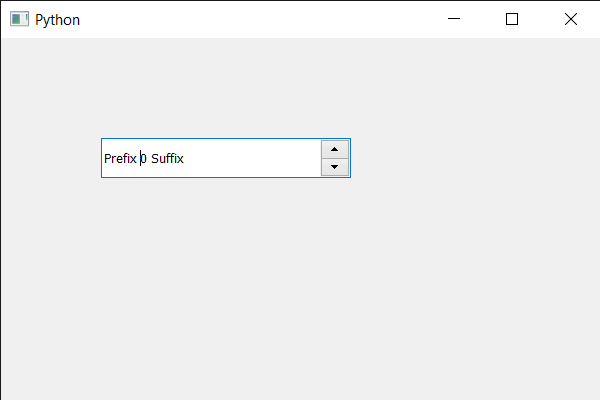# PyQt5 QSpinBox – Setting Properties

• Last Updated : 19 May, 2020

In this article we will see how we can set properties to the spin box, properties are the information added by the developer to tell about the properties of the spin box, for example if spin box has a ability to loop the values, developer will add this property so that other developer can see this and get to know about the property.

In order to do this we use setProperty method

Syntax : spin_box.setProperty(property, value)

Argument : It takes two argument one is string and other is integer

Action performed : It add this property to the spin box

Below is the implementation

 `# importing libraries``from` `PyQt5.QtWidgets ``import` `*` `from` `PyQt5 ``import` `QtCore, QtGui``from` `PyQt5.QtGui ``import` `*` `from` `PyQt5.QtCore ``import` `*` `import` `sys`` ` ` ` `class` `Window(QMainWindow):`` ` `    ``def` `__init__(``self``):``        ``super``().__init__()`` ` `        ``# setting title``        ``self``.setWindowTitle(``"Python "``)`` ` `        ``# setting geometry``        ``self``.setGeometry(``100``, ``100``, ``600``, ``400``)`` ` `        ``# calling method``        ``self``.UiComponents()`` ` `        ``# showing all the widgets``        ``self``.show()`` ` `        ``# method for widgets``    ``def` `UiComponents(``self``):``        ``# creating spin box``        ``self``.spin ``=` `QSpinBox(``self``)`` ` `        ``# setting geometry to spin box``        ``self``.spin.setGeometry(``100``, ``100``, ``250``, ``40``)`` ` `        ``# setting range to the spin box``        ``self``.spin.setRange(``0``, ``999999``)`` ` `        ``# setting prefix to spin``        ``self``.spin.setPrefix(``"Prefix "``)`` ` `        ``# setting suffix to spin``        ``self``.spin.setSuffix(``" Suffix"``)`` ` `        ``# adding property to the spin box``        ``self``.spin.setProperty(``"Dynamic"``, ``1``)``         ` `        ``# adding property to the spin box``        ``self``.spin.setProperty(``"Has suffix"``, ``1``)`` ` ` ` ` ` `# create pyqt5 app``App ``=` `QApplication(sys.argv)`` ` `# create the instance of our Window``window ``=` `Window()``window.spin.setFocus()``# start the app``sys.exit(App.``exec``())`

Output :My Personal Notes arrow_drop_up